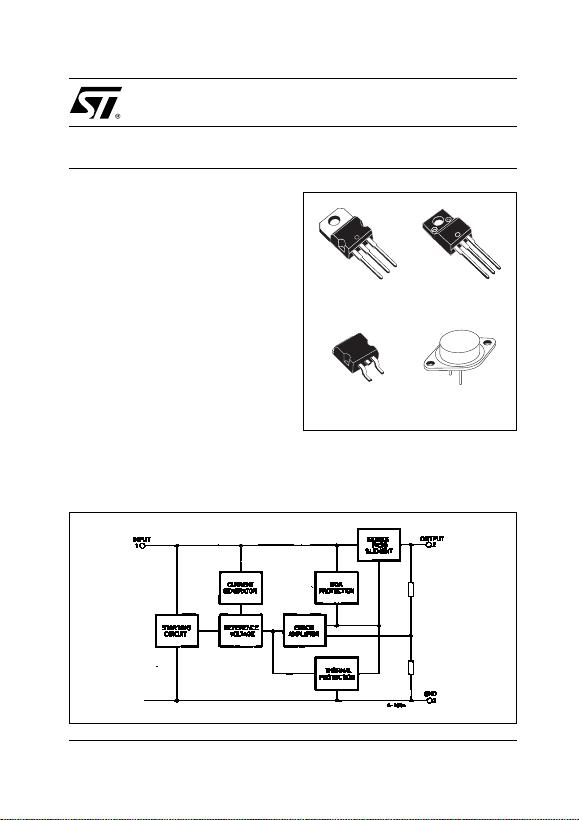1/29February 2003
OUTPUT CURRENT TO 1.5A
OUTPUT VOLTAGES OF 5; 5.2; 6; 8; 8.5; 9;
12; 15; 18; 24V
SHORT CIRCUIT PROTECTION
OUTPUT TRANSITION SOA PROTECTION
DESCRIPTION
The L7800 series of three-terminal positive
regulators is available in TO-220, TO-220FP,
TO-3 and D2PAK packages and several fixed
output voltages, making it useful in a wide range of
applications. These regulators can provide local
on-card regulation, eliminating the distribution
problems associated with single point regulation.
Each type employs internal current limiting,
thermal shut-down and safe area protection,
making it essentially indestructible. If adequate
heat sinking is provided, they can deliver over 1A
output current. Although designed primarily as
fixed voltage regulators, these devices can be
used with external components to obtain
L7800
SERIES
POSITIVE VOLTAGE REGULATORS
SCHEMATIC DIAGRAM
TO-220
D2PAK
TO-220FP
TO-3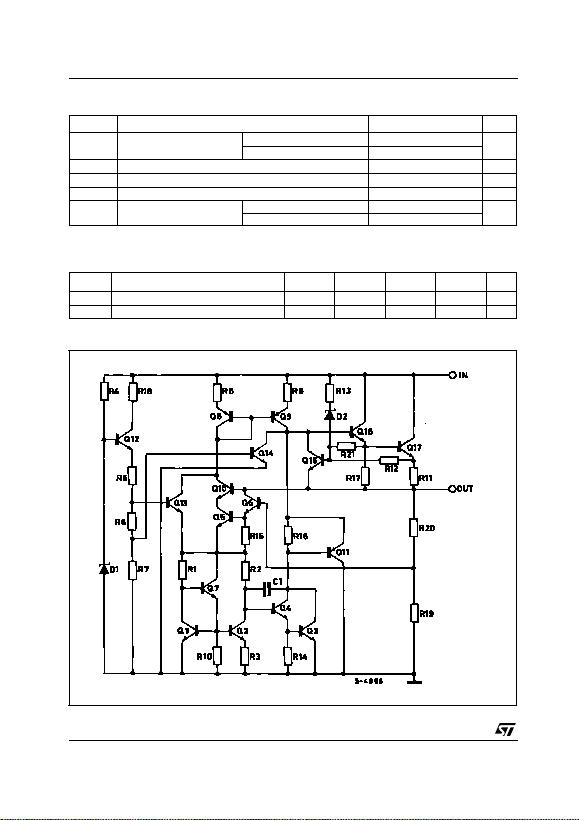L7800 SERIES
2/29
ABSOLUTE MAXIMUM RATINGS
Absolute Maximum Ratings are those values beyond which damage to the device may occur. Functional operation under these condition is
not implied.
THERMAL DATA
SCHEMATIC DIAGRAM
Symbol Parameter² Value Unit
VIDC Input Voltage for VO= 5 to 18V 35 V
for VO= 20, 24V 40
IOOutput Current Internally Limited
Ptot Power Dissipation Internally Limited
Tstg Storage Temperature Range -65 to 150 °C
Top OperatingJunctionTemperature
Range for L7800 -55 to 150 °C
for L7800C 0 to 150
Symbol Parameter D2PAK TO-220 TO-220FP TO-3 Unit
Rthj-case Thermal Resistance Junction-case Max 3554°C/W
R
thj-amb Thermal Resistance Junction-ambient Max 62.5 50 60 35 °C/W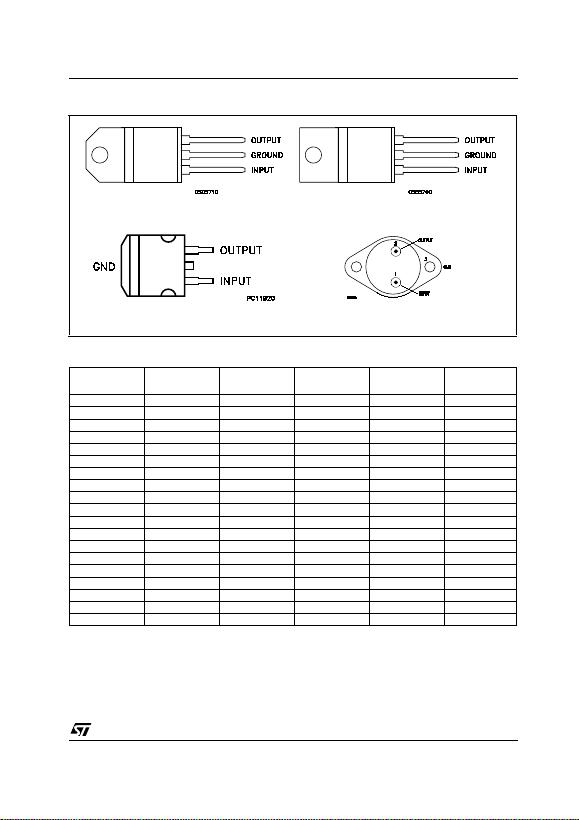L7800 SERIES
3/29
CONNECTION DIAGRAM (top view)
ORDERING CODES
(*) Available in Tape & Reel with the suffix "-TR".
TYPE TO-220 D2PAK (*) TO-220FP TO-3 OUTPUT
VOLTAGE
L7805 L7805T 5 V
L7805C L7805CV L7805CD2T L7805CP L7805CT 5 V
L7852C L7852CV L7852CD2T L7852CP L7852CT 5.2 V
L7806 L7806T 6 V
L7806C L7806CV L7806CD2T L7806CP L7806CT 6 V
L7808 L7808T 8 V
L7808C L7808CV L7808CD2T L7808CP L7808CT 8 V
L7885C L7885CV L7885CD2T L7885CP L7885CT 8.5 V
L7809C L7809CV L7809CD2T L7809CP L7809CT 9 V
L7812 L7812T 12 V
L7812C L7812CV L7812CD2T L7812CP L7812CT 12 V
L7815 L7815T 15 V
L7815C L7815CV L7815CD2T L7815CP L7815CT 15 V
L7818 L7818T 18 V
L7818C L7818CV L7818CD2T L7818CP L7818CT 18 V
L7820 L7820T 20 V
L7820C L7820CV L7820CD2T L7820CP L7820CT 20 V
L7824 L7824T 24 V
L7824C L7824CV L7824CD2T L7824CP L7824CT 24 V
TO-220 TO-220FP
TO-3D2PAK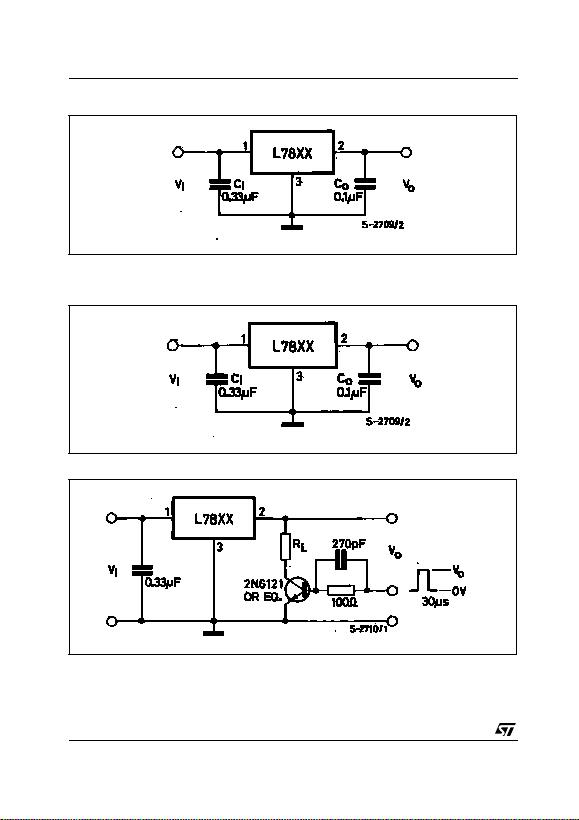L7800 SERIES
4/29
APPLICATION CIRCUITS
TEST CIRCUITS
Figure 1 : DC Parameter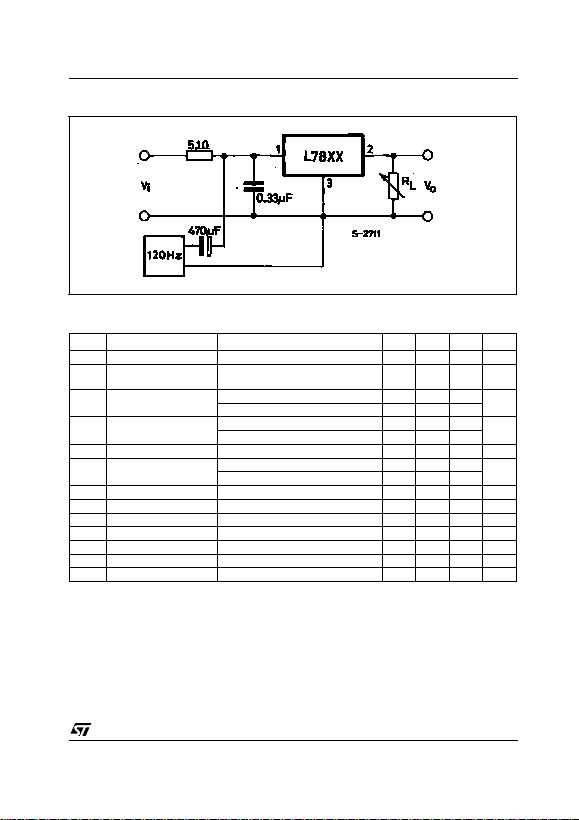L7800 SERIES
5/29
Figure 3 : Ripple Rejection
ELECTRICAL CHARACTERISTICS OF L7805 (refer to the test circuits, TJ= -55 to 15C, VI= 10V,
IO=500mA,C
I=0.33µF,C
O= 0.1 µF unless otherwise specified).
(*) Load and line regulation are specified at constant junction temperature. Changes in VOdue to heating effects must be taken into account
separately. Pulse testing with low duty cycle is used.
Symbol Parameter Test Conditions Min. Typ. Max. Unit
VOOutput Voltage TJ= 25°C 4.8 5 5.2 V
VOOutput Voltage IO=5mAto1A P
O15W
VI= 8 to 20 V 4.65 5 5.35 V
VO(*) Line Regulation VI=7to25V T
J= 25°C 3 50 mV
VI=8to12V T
J= 25°C 1 25
J= 25°C 100 mV
IO= 250 to 750 mA TJ= 25°C 25
IdQuiescent Current TJ= 25°C 6 mA
IdQuiescent Current Change IO=5mAto1A 0.5 mA
V
I= 8 to 25 V 0.8
VO/T Output Voltage Drift IO= 5 mA 0.6 mV/°C
eN Output Noise Voltage B =10Hz to 100KHz TJ= 25°C 40 µV/VO
SVR Supply Voltage Rejection VI= 8 to 18 V f = 120Hz 68 dB
VdDropout Voltage IO=1A T
J= 25°C 2 2.5 V
ROOutput Resistance f = 1 KHz 17 m
Isc Short Circuit Current VI=35V T
J= 25°C 0.75 1.2 A
Iscp Short Circuit Peak Current TJ= 25°C 1.3 2.2 3.3 A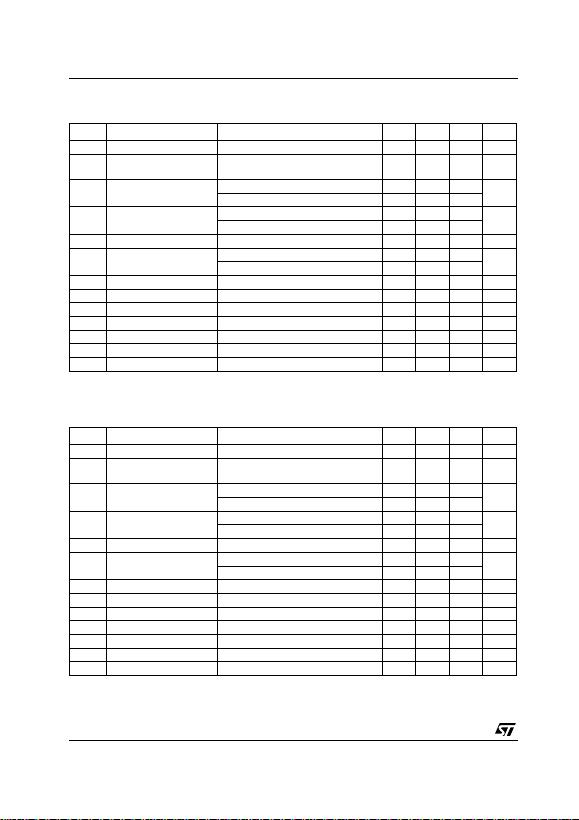L7800 SERIES
6/29
ELECTRICAL CHARACTERISTICS OF L7806 (refer to the test circuits, TJ= -55 to 150°C, VI= 11V,
IO=500mA,C
I=0.33µF,C
O= 0.1 µF unless otherwise specified).
(*) Load and line regulation are specified at constant junction temperature. Changes in VOdue to heating effects must be taken into account
separately. Pulse testing with low duty cycle is used.
ELECTRICAL CHARACTERISTICS OF L7808 (refer to the test circuits, TJ= -55 to 150°C, VI= 14V,
IO=500mA,C
I=0.33µF,C
O= 0.1 µF unless otherwise specified).
(*) Load and line regulation are specified at constant junction temperature. Changes in VOdue to heating effects must be taken into account
separately. Pulse testing with low duty cycle is used.
Symbol Parameter Test Conditions Min. Typ. Max. Unit
VOOutput Voltage TJ= 25°C 5.75 6 6.25 V
VOOutput Voltage IO=5mAto1A P
O15W
VI= 9 to 21 V 5.65 6 6.35 V
VO(*) Line Regulation VI=8to25V T
J= 25°C 60 mV
VI=9to13V T
J= 25°C 30
J= 25°C 100 mV
IO= 250 to 750 mA TJ= 25°C 30
IdQuiescent Current TJ= 25°C 6 mA
IdQuiescent Current Change IO=5mAto1A 0.5 mA
V
I= 9 to 25 V 0.8
VO/T Output Voltage Drift IO= 5 mA 0.7 mV/°C
eN Output Noise Voltage B =10Hz to 100KHz TJ= 25°C 40 µV/VO
SVR Supply Voltage Rejection VI= 9 to 19 V f = 120Hz 65 dB
VdDropout Voltage IO=1A T
J= 25°C 2 2.5 V
ROOutput Resistance f = 1 KHz 19 m
Isc Short Circuit Current VI=35V T
J= 25°C 0.75 1.2 A
Iscp Short Circuit Peak Current TJ= 25°C 1.3 2.2 3.3 A
Symbol Parameter Test Conditions Min. Typ. Max. Unit
VOOutput Voltage TJ= 25°C 7.7 8 8.3 V
VOOutput Voltage IO=5mAto1A P
O15W
VI= 11.5 to 23 V 7.6 8 8.4 V
VO(*) Line Regulation VI= 10.5 to 25 V TJ= 25°C 80 mV
VI=11to17V T
J= 25°C 40
J= 25°C 100 mV
IO= 250 to 750 mA TJ= 25°C 40
IdQuiescent Current TJ= 25°C 6 mA
IdQuiescent Current Change IO=5mAto1A 0.5 mA
V
I= 11.5 to 25 V 0.8
VO/T Output Voltage Drift IO= 5 mA 1 mV/°C
eN Output Noise Voltage B =10Hz to 100KHz TJ= 25°C 40 µV/VO
SVR Supply Voltage Rejection VI= 11.5 to 21.5 V f = 120Hz 62 dB
VdDropout Voltage IO=1A T
J= 25°C 2 2.5 V
ROOutput Resistance f = 1 KHz 16 m
Isc Short Circuit Current VI=35V T
J= 25°C 0.75 1.2 A
Iscp Short Circuit Peak Current TJ= 25°C 1.3 2.2 3.3 A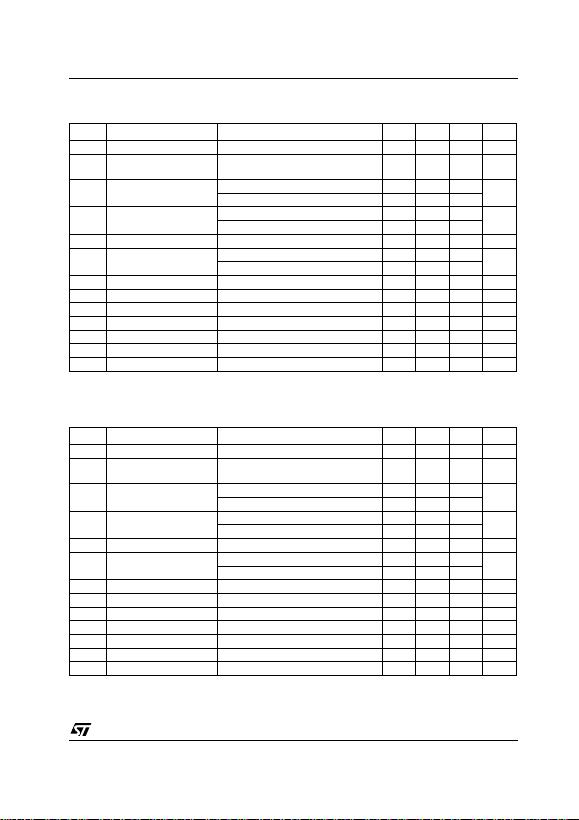L7800 SERIES
7/29
ELECTRICAL CHARACTERISTICS OF L7812 (refer to the test circuits, TJ= -55 to 150°C, VI= 19V,
IO=500mA,C
I=0.33µF,C
O= 0.1 µF unless otherwise specified).
(*) Load and line regulation are specified at constant junction temperature. Changes in VOdue to heating effects must be taken into account
separately. Pulse testing with low duty cycle is used.
ELECTRICAL CHARACTERISTICS OF L7815 (refer to the test circuits, TJ= -55 to 150°C, VI= 23V,
IO=500mA,C
I=0.33µF,C
O= 0.1 µF unless otherwise specified).
(*) Load and line regulation are specified at constant junction temperature. Changes in VOdue to heating effects must be taken into account
separately. Pulse testing with low duty cycle is used.
Symbol Parameter Test Conditions Min. Typ. Max. Unit
VOOutput Voltage TJ= 25°C 11.5 12 12.5 V
VOOutput Voltage IO=5mAto1A P
O15W
VI= 15.5 to 27 V 11.4 12 12.6 V
VO(*) Line Regulation VI= 14.5 to 30 V TJ= 25°C 120 mV
VI=16to22V T
J= 25°C 60
J= 25°C 100 mV
IO= 250 to 750 mA TJ= 25°C 60
IdQuiescent Current TJ= 25°C 6 mA
IdQuiescent Current Change IO=5mAto1A 0.5 mA
V
I=15to30V 0.8
V
O
/T Output Voltage Drift IO= 5 mA 1.5 mV/°C
eN Output Noise Voltage B =10Hz to 100KHz TJ= 25°C 40 µV/VO
SVR Supply Voltage Rejection VI= 15 to 25 V f = 120Hz 61 dB
VdDropout Voltage IO=1A T
J= 25°C 2 2.5 V
ROOutput Resistance f = 1 KHz 18 m
Isc Short Circuit Current VI=35V T
J= 25°C 0.75 1.2 A
Iscp Short Circuit Peak Current TJ= 25°C 1.3 2.2 3.3 A
Symbol Parameter Test Conditions Min. Typ. Max. Unit
VOOutput Voltage TJ= 25°C 14.4 15 15.6 V
VOOutput Voltage IO=5mAto1A P
O15W
VI= 18.5 to 30 V 14.25 15 15.75 V
VO(*) Line Regulation VI= 17.5 to 30 V TJ= 25°C 150 mV
VI=20to26V T
J= 25°C 75
J= 25°C 150 mV
IO= 250 to 750 mA TJ= 25°C 75
IdQuiescent Current TJ= 25°C 6 mA
IdQuiescent Current Change IO=5mAto1A 0.5 mA
V
I= 18.5 to 30 V 0.8
VO/T Output Voltage Drift IO= 5 mA 1.8 mV/°C
eN Output Noise Voltage B =10Hz to 100KHz TJ= 25°C 40 µV/VO
SVR Supply Voltage Rejection VI= 18.5 to 28.5 V f = 120Hz 60 dB
VdDropout Voltage IO=1A T
J= 25°C 2 2.5 V
ROOutput Resistance f = 1 KHz 19 m
Isc Short Circuit Current VI=35V T
J= 25°C 0.75 1.2 A
Iscp Short Circuit Peak Current TJ= 25°C 1.3 2.2 3.3 A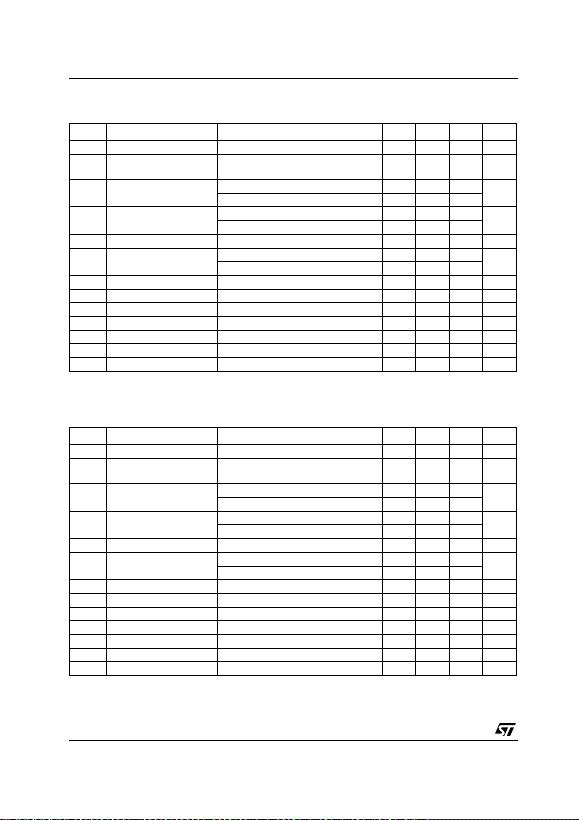L7800 SERIES
8/29
ELECTRICAL CHARACTERISTICS OF L7818 (refer to the test circuits, TJ= -55 to 150°C, VI= 26V,
IO=500mA,C
I=0.33µF,C
O= 0.1 µF unless otherwise specified).
(*) Load and line regulation are specified at constant junction temperature. Changes in VOdue to heating effects must be taken into account
separately. Pulse testing with low duty cycle is used.
ELECTRICAL CHARACTERISTICS OF L7820 (refer to the test circuits, TJ= -55 to 150°C, VI= 28V,
IO=500mA,C
I=0.33µF,C
O= 0.1 µF unless otherwise specified).
(*) Load and line regulation are specified at constant junction temperature. Changes in VOdue to heating effects must be taken into account
separately. Pulse testing with low duty cycle is used.
Symbol Parameter Test Conditions Min. Typ. Max. Unit
VOOutput Voltage TJ= 25°C 17.3 18 18.7 V
VOOutput Voltage IO=5mAto1A P
O15W
VI=22to33V 17.1 18 18.9 V
VO(*) Line Regulation VI=21to33V T
J= 25°C 180 mV
VI=24to30V T
J= 25°C 90
J= 25°C 180 mV
IO= 250 to 750 mA TJ= 25°C 90
IdQuiescent Current TJ= 25°C 6 mA
IdQuiescent Current Change IO=5mAto1A 0.5 mA
V
I=22to33V 0.8
V
O
/T Output Voltage Drift IO= 5 mA 2.3 mV/°C
eN Output Noise Voltage B =10Hz to 100KHz TJ= 25°C 40 µV/VO
SVR Supply Voltage Rejection VI= 22 to 32 V f = 120Hz 59 dB
VdDropout Voltage IO=1A T
J= 25°C 2 2.5 V
ROOutput Resistance f = 1 KHz 22 m
Isc Short Circuit Current VI=35V T
J= 25°C 0.75 1.2 A
Iscp Short Circuit Peak Current TJ= 25°C 1.3 2.2 3.3 A
Symbol Parameter Test Conditions Min. Typ. Max. Unit
VOOutput Voltage TJ= 25°C 19.2 20 20.8 V
VOOutput Voltage IO=5mAto1A P
O15W
VI=24to35V 19 20 21 V
VO(*) Line Regulation VI= 22.5 to 35 V TJ= 25°C 200 mV
VI=26to32V T
J= 25°C 100
J= 25°C 200 mV
IO= 250 to 750 mA TJ= 25°C 100
IdQuiescent Current TJ= 25°C 6 mA
IdQuiescent Current Change IO=5mAto1A 0.5 mA
V
I=24to35V 0.8
V
O
/T Output Voltage Drift IO= 5 mA 2.5 mV/°C
eN Output Noise Voltage B =10Hz to 100KHz TJ= 25°C 40 µV/VO
SVR Supply Voltage Rejection VI= 24 to 35 V f = 120Hz 58 dB
VdDropout Voltage IO=1A T
J= 25°C 2 2.5 V
ROOutput Resistance f = 1 KHz 24 m
Isc Short Circuit Current VI=35V T
J= 25°C 0.75 1.2 A
Iscp Short Circuit Peak Current TJ= 25°C 1.3 2.2 3.3 A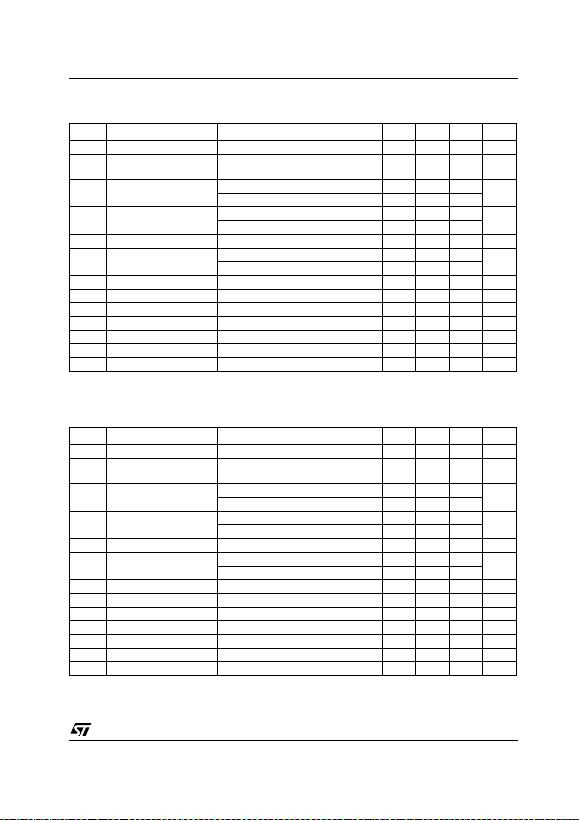L7800 SERIES
9/29
ELECTRICAL CHARACTERISTICS OF L7824 (refer to the test circuits, TJ= -55 to 150°C, VI= 33V,
IO=500mA,C
I=0.33µF,C
O= 0.1 µF unless otherwise specified).
(*) Load and line regulation are specified at constant junction temperature. Changes in VOdue to heating effects must be taken into account
separately. Pulse testing with low duty cycle is used.
ELECTRICAL CHARACTERISTICS OF L7805C (refer to the test circuits, TJ= -55 to 150°C, VI= 10V,
IO=500mA,C
I=0.33µF,C
O= 0.1 µF unless otherwise specified).
(*) Load and line regulation are specified at constant junction temperature. Changes in VOdue to heating effects must be taken into account
separately. Pulse testing with low duty cycle is used.
Symbol Parameter Test Conditions Min. Typ. Max. Unit
VOOutput Voltage TJ=25°C 232425 V
V
O
Output Voltage IO=5mAto1A P
O15W
VI=28to38V 22.8 24 25.2 V
VO(*) Line Regulation VI=27to38V T
J= 25°C 240 mV
VI=30to36V T
J= 25°C 120
J= 25°C 240 mV
IO= 250 to 750 mA TJ= 25°C 120
IdQuiescent Current TJ= 25°C 6 mA
IdQuiescent Current Change IO=5mAto1A 0.5 mA
V
I=28to38V 0.8
V
O
/T Output Voltage Drift IO= 5 mA 3 mV/°C
eN Output Noise Voltage B =10Hz to 100KHz TJ= 25°C 40 µV/VO
SVR Supply Voltage Rejection VI= 28 to 38 V f = 120Hz 56 dB
VdDropout Voltage IO=1A T
J= 25°C 2 2.5 V
ROOutput Resistance f = 1 KHz 28 m
Isc Short Circuit Current VI=35V T
J= 25°C 0.75 1.2 A
Iscp Short Circuit Peak Current TJ= 25°C 1.3 2.2 3.3 A
Symbol Parameter Test Conditions Min. Typ. Max. Unit
VOOutput Voltage TJ= 25°C 4.8 5 5.2 V
VOOutput Voltage IO=5mAto1A P
O15W
VI= 7 to 20 V 4.75 5 5.25 V
VO(*) Line Regulation VI=7to25V T
J= 25°C 3 100 mV
VI=8to12V T
J= 25°C 1 50
J= 25°C 100 mV
IO= 250 to 750 mA TJ= 25°C 50
IdQuiescent Current TJ= 25°C 8 mA
IdQuiescent Current Change IO=5mAto1A 0.5 mA
V
I= 7 to 25 V 0.8
VO/T Output Voltage Drift IO= 5 mA -1.1 mV/°C
eN Output Noise Voltage B =10Hz to 100KHz TJ= 25°C 40 µV/VO
SVR Supply Voltage Rejection VI= 8 to 18 V f = 120Hz 62 dB
VdDropout Voltage IO=1A T
J= 25°C 2 V
ROOutput Resistance f = 1 KHz 17 m
Isc Short Circuit Current VI=35V T
J= 25°C 0.75 A
Iscp Short Circuit Peak Current TJ= 25°C 2.2 A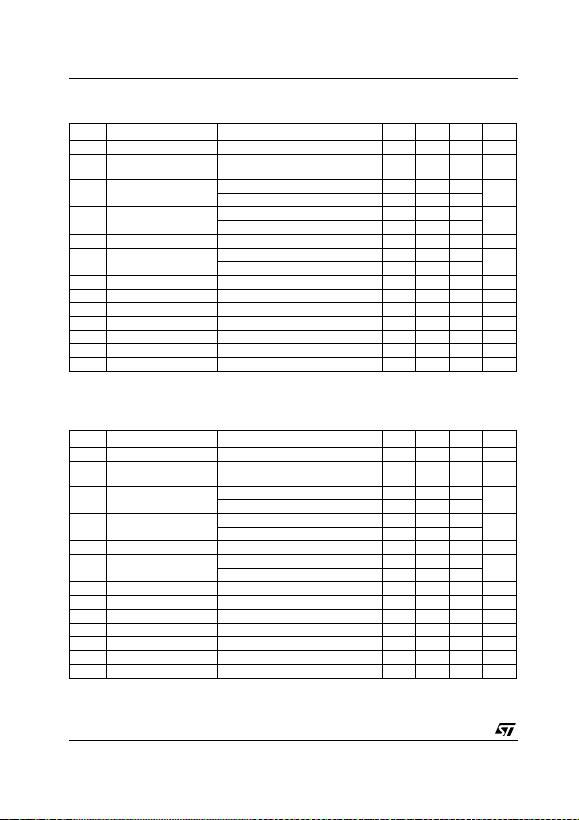L7800 SERIES
10/29
ELECTRICAL CHARACTERISTICS OF L7852C (refer to the test circuits, TJ= -55 to 150°C, VI= 10V,
IO=500mA,C
I=0.33µF,C
O= 0.1 µF unless otherwise specified).
(*) Load and line regulation are specified at constant junction temperature. Changes in VOdue to heating effects must be taken into account
separately. Pulse testing with low duty cycle is used.
ELECTRICAL CHARACTERISTICS OF L7806C (refer to the test circuits, TJ= -55 to 150°C, VI= 11V,
IO=500mA,C
I=0.33µF,C
O= 0.1 µF unless otherwise specified).
(*) Load and line regulation are specified at constant junction temperature. Changes in VOdue to heating effects must be taken into account
separately. Pulse testing with low duty cycle is used.
Symbol Parameter Test Conditions Min. Typ. Max. Unit
VOOutput Voltage TJ= 25°C 5.0 5.2 5.4 V
VOOutput Voltage IO=5mAto1A P
O15W
VI= 8 to 20 V 4.95 5.2 5.45 V
VO(*) Line Regulation VI=7to25V T
J= 25°C 3 105 mV
VI=8to12V T
J= 25°C 1 52
J= 25°C 105 mV
IO= 250 to 750 mA TJ= 25°C 52
IdQuiescent Current TJ= 25°C 8 mA
IdQuiescent Current Change IO=5mAto1A 0.5 mA
V
I= 7 to 25 V 1.3
VO/T Output Voltage Drift IO= 5 mA -1 mV/°C
eN Output Noise Voltage B =10Hz to 100KHz TJ= 25°C 42 µV/VO
SVR Supply Voltage Rejection VI= 8 to 18 V f = 120Hz 61 dB
VdDropout Voltage IO=1A T
J= 25°C 2 V
ROOutput Resistance f = 1 KHz 17 m
Isc Short Circuit Current VI=35V T
J= 25°C 0.75 A
Iscp Short Circuit Peak Current TJ= 25°C 2.2 A
Symbol Parameter Test Conditions Min. Typ. Max. Unit
VOOutput Voltage TJ= 25°C 5.75 6 6.25 V
VOOutput Voltage IO=5mAto1A P
O15W
VI= 8 to 21 V 5.7 6 6.3 V
VO(*) Line Regulation VI=8to25V T
J= 25°C 120 mV
VI=9to13V T
J= 25°C 60
J= 25°C 120 mV
IO= 250 to 750 mA TJ= 25°C 60
IdQuiescent Current TJ= 25°C 8 mA
IdQuiescent Current Change IO=5mAto1A 0.5 mA
V
I= 8 to 25 V 1.3
VO/T Output Voltage Drift IO= 5 mA -0.8 mV/°C
eN Output Noise Voltage B =10Hz to 100KHz TJ= 25°C 45 µV/VO
SVR Supply Voltage Rejection VI= 9 to 19 V f = 120Hz 59 dB
VdDropout Voltage IO=1A T
J= 25°C 2 V
ROOutput Resistance f = 1 KHz 19 m
Isc Short Circuit Current VI=35V T
J= 25°C 0.55 A
Iscp Short Circuit Peak Current TJ= 25°C 2.2 A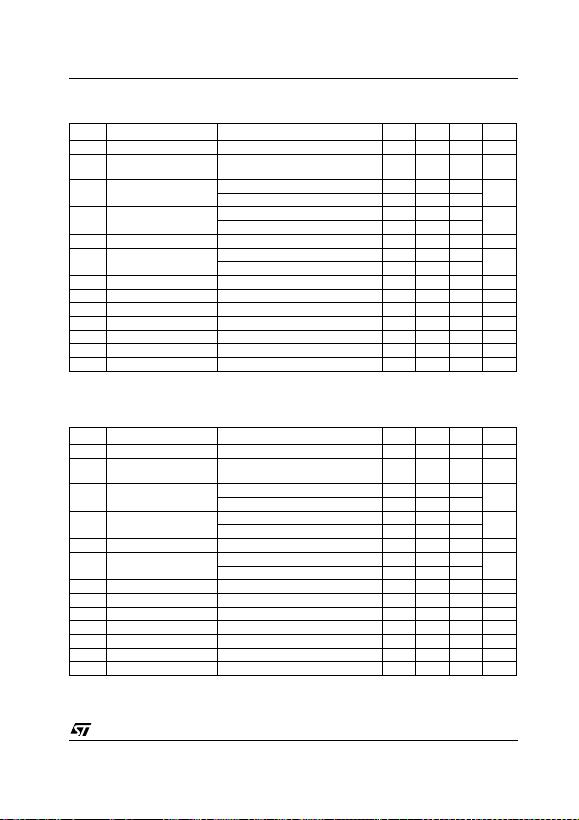L7800 SERIES
11/29
ELECTRICAL CHARACTERISTICS OF L7808C (refer to the test circuits, TJ= -55 to 150°C, VI= 14V,
IO=500mA,C
I=0.33µF,C
O= 0.1 µF unless otherwise specified).
(*) Load and line regulation are specified at constant junction temperature. Changes in VOdue to heating effects must be taken into account
separately. Pulse testing with low duty cycle is used.
ELECTRICAL CHARACTERISTICS OF L7885C (refer to the test circuits, TJ= -55 to 150°C, VI= 14.5V,
IO=500mA,C
I=0.33µF,C
O= 0.1 µF unless otherwise specified).
(*) Load and line regulation are specified at constant junction temperature. Changes in VOdue to heating effects must be taken into account
separately. Pulse testing with low duty cycle is used.
Symbol Parameter Test Conditions Min. Typ. Max. Unit
VOOutput Voltage TJ= 25°C 7.7 8 8.3 V
VOOutput Voltage IO=5mAto1A P
O15W
VI= 10.5 to 25 V 7.6 8 8.4 V
VO(*) Line Regulation VI= 10.5 to 25 V TJ= 25°C 160 mV
VI=11to17V T
J= 25°C 80
J= 25°C 160 mV
IO= 250 to 750 mA TJ= 25°C 80
IdQuiescent Current TJ= 25°C 8 mA
IdQuiescent Current Change IO=5mAto1A 0.5 mA
V
I= 10.5 to 25 V 1
VO/T Output Voltage Drift IO= 5 mA -0.8 mV/°C
eN Output Noise Voltage B =10Hz to 100KHz TJ= 25°C 52 µV/VO
SVR Supply Voltage Rejection VI= 11.5 to 21.5 V f = 120Hz 56 dB
VdDropout Voltage IO=1A T
J= 25°C 2 V
ROOutput Resistance f = 1 KHz 16 m
Isc Short Circuit Current VI=35V T
J= 25°C 0.45 A
Iscp Short Circuit Peak Current TJ= 25°C 2.2 A
Symbol Parameter Test Conditions Min. Typ. Max. Unit
VOOutput Voltage TJ= 25°C 8.2 8.5 8.8 V
VOOutput Voltage IO=5mAto1A P
O15W
VI=11to26V 8.1 8.5 8.9 V
VO(*) Line Regulation VI=11to27V T
J= 25°C 160 mV
VI= 11.5 to 17.5 V TJ= 25°C 80
J= 25°C 160 mV
IO= 250 to 750 mA TJ= 25°C 80
IdQuiescent Current TJ= 25°C 8 mA
IdQuiescent Current Change IO=5mAto1A 0.5 mA
V
I=11to27V 1
V
O
/T Output Voltage Drift IO= 5 mA -0.8 mV/°C
eN Output Noise Voltage B =10Hz to 100KHz TJ= 25°C 55 µV/VO
SVR Supply Voltage Rejection VI= 12 to 22 V f = 120Hz 56 dB
VdDropout Voltage IO=1A T
J= 25°C 2 V
ROOutput Resistance f = 1 KHz 16 m
Isc Short Circuit Current VI=35V T
J= 25°C 0.45 A
Iscp Short Circuit Peak Current TJ= 25°C 2.2 A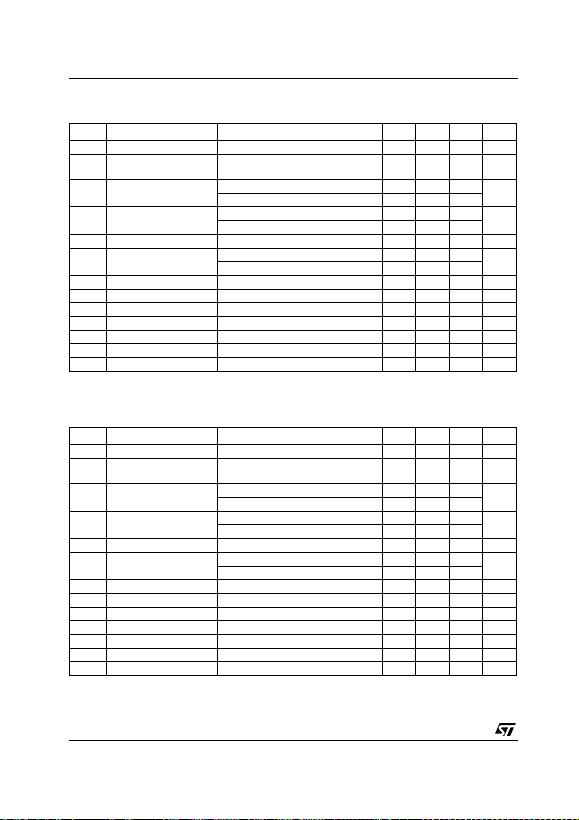L7800 SERIES
12/29
ELECTRICAL CHARACTERISTICS OF L7809C (refer to the test circuits, TJ= -55 to 150°C, VI= 15V,
IO=500mA,C
I=0.33µF,C
O= 0.1 µF unless otherwise specified).
(*) Load and line regulation are specified at constant junction temperature. Changes in VOdue to heating effects must be taken into account
separately. Pulse testing with low duty cycle is used.
ELECTRICAL CHARACTERISTICS OF L7812C (refer to the test circuits, TJ= -55 to 150°C, VI= 19V,
IO=500mA,C
I=0.33µF,C
O= 0.1 µF unless otherwise specified).
(*) Load and line regulation are specified at constant junction temperature. Changes in VOdue to heating effects must be taken into account
separately. Pulse testing with low duty cycle is used.
Symbol Parameter Test Conditions Min. Typ. Max. Unit
VOOutput Voltage TJ= 25°C 8.65 9 9.35 V
VOOutput Voltage IO=5mAto1A P
O15W
VI= 11.5 to 26 V 8.55 9 9.45 V
VO(*) Line Regulation VI= 11.5 to 26 V TJ= 25°C 180 mV
VI=12to18V T
J= 25°C 90
J= 25°C 180 mV
IO= 250 to 750 mA TJ= 25°C 90
IdQuiescent Current TJ= 25°C 8 mA
IdQuiescent Current Change IO=5mAto1A 0.5 mA
V
I= 11.5 to 26 V 1
VO/T Output Voltage Drift IO= 5 mA -1 mV/°C
eN Output Noise Voltage B =10Hz to 100KHz TJ= 25°C 70 µV/VO
SVR Supply Voltage Rejection VI= 12 to 23 V f = 120Hz 55 dB
VdDropout Voltage IO=1A T
J= 25°C 2 V
ROOutput Resistance f = 1 KHz 17 m
Isc Short Circuit Current VI=35V T
J= 25°C 0.40 A
Iscp Short Circuit Peak Current TJ= 25°C 2.2 A
Symbol Parameter Test Conditions Min. Typ. Max. Unit
VOOutput Voltage TJ= 25°C 11.5 12 12.5 V
VOOutput Voltage IO=5mAto1A P
O15W
VI= 14.5 to 27 V 11.4 12 12.6 V
VO(*) Line Regulation VI= 14.5 to 30 V TJ= 25°C 240 mV
VI=16to22V T
J= 25°C 120
J= 25°C 240 mV
IO= 250 to 750 mA TJ= 25°C 120
IdQuiescent Current TJ= 25°C 8 mA
IdQuiescent Current Change IO=5mAto1A 0.5 mA
V
I= 14.5 to 30 V 1
VO/T Output Voltage Drift IO= 5 mA -1 mV/°C
eN Output Noise Voltage B =10Hz to 100KHz TJ= 25°C 75 µV/VO
SVR Supply Voltage Rejection VI= 15 to 25 V f = 120Hz 55 dB
VdDropout Voltage IO=1A T
J= 25°C 2 V
ROOutput Resistance f = 1 KHz 18 m
Isc Short Circuit Current VI=35V T
J= 25°C 0.35 A
Iscp Short Circuit Peak Current TJ= 25°C 2.2 A